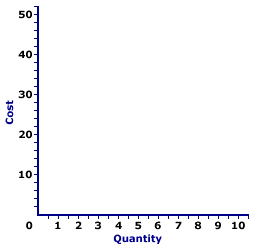Saturday  March 25, 2023
 AmosWEB means Economics with a Touch of Whimsy!ZERO BOND: Also termed a zero coupon bond, a bond that does not pay interest, in which the return is generated by the difference between the purchase price and the face value paid at maturity. Because they do not pay interest, zero bonds are sold at a discount. For example, a \$10,000 zero bond that matures in one year, would generate a 10% return if it sold at a discount of \$9,000.TOTAL COST CURVES:

The total cost of producing a good can be represented by three related curves, total cost curve, total variable cost curve, and total fixed cost curve. The total cost curve is the vertical summation of the total variable cost curve and the total fixed cost curve.
Total Cost CurvesThe three curves reflecting that total cost that is related to the short-run production are the total fixed cost curve, the total variable cost curve, and the total cost curve. The exhibit to the right can be used to display the three total cost curves.
• Total Fixed Cost Curve: The total fixed cost (TFC) curve is a horizontal line. Click the [TFC] button to display the curve for Wacky Willy Stuffed Amigos production. Total fixed cost is equal to \$3 and does not change with the quantity of output produced, thus the TFC curve is a flat, horizontal line.

• Total Variable Cost Curve: The total variable cost (TVC) curve is a positively-sloped line that reflects increasing then decreasing marginal returns. Click the [TVC] button to add this curve. The TVC curve emerges from the origin with a relatively steep slope, flattens, then becomes increasingly steeper. In the context of Wacky Willy Stuffed Amigos, the TVC for one Stuffed Amigo is \$5, this then rises to \$8 for two Stuffed Amigos, \$10 for three Stuffed Amigos, etc., finally reaching \$43 for ten Stuffed Amigos.

• Total Cost Curve: The total cost (TC) curve can be derived as the vertical summation of the TVC and TFC curves. In other words, the TC curve can be found by shifting the TVC vertically by the amount of TFC. This means that the shape of the TC curve is identical to that of the TVC. The two curves have identical slopes for each quantity of output. For Wacky Willy Stuffed Amigos production, the vertical difference between the TC and TVC curves is exactly \$3.00, the value of TFC. Click the [TC] button to identify this curve.
An important conclusion from this derivation of the TC curve is that the vertical distance between TC and TVC curves is the same at ALL output quantities. The reason, of course, is that this vertical distance IS total fixed cost. Because total fixed cost is constant, the vertical distance is constant.

From a geometric perspective, a constant gap between two lines means that the lines are parallel. While parallel lines are commonly presented as straight lines, two curved lines like the TC and TVC can also be parallel so long as they do not intersect and their distance remains constant.

This further implies that the slopes of the TC and TVC curves are identical at each and every output quantity. In fact, it is acceptable to think of the TC curve as the result of vertically shifting the TVC upward by the amount of total fixed cost. In other words, the TC and TVC curves are essentially the same curve, the TC curve just happens to be a little bit higher in the diagram, higher by the amount of total fixed cost.

However, in that the slope of the TC curve is marginal cost, the slope of the TVC curve is also marginal cost. Marginal cost, the increment of total cost resulting from a change in quantity, can be derived using either total cost or total variable cost. This point should be evident by noting that any quantity-induced change in total cost must come from total variable cost. Total fixed cost is fixed, it does not change. As such, marginal cost, the change in total cost, is not affected by total fixed cost.

 <= TOTAL COST CURVE TOTAL FACTOR COST =>Recommended Citation:

TOTAL COST CURVES, AmosWEB Encyclonomic WEB*pedia, http://www.AmosWEB.com, AmosWEB LLC, 2000-2023. [Accessed: March 25, 2023].

Check Out These Related Terms...

Or For A Little Background...

And For Further Study...
Search Again?BLUE PLACIDOLA[What's This?] Today, you are likely to spend a great deal of time wandering around the shopping mall trying to buy either a wall poster commemorating the first day of spring or a lazy Susan for you dining room table. Be on the lookout for gnomes hiding in cypress trees.Your Complete ScopeThe average length of a "business lunch" is about 36 minutes."Success is the ability to go from one failure to another with no loss of enthusiasm."-- Sir Winston ChurchillVSEVancouver Stock Exchange (Canada)A PEDestrian's Guide Xtra CreditTell us what you think about AmosWEB. Like what you see? Have suggestions for improvements? Let us know. Click the User Feedback link.| | | | | | | | | | |
| | | |

Thanks for visiting AmosWEB